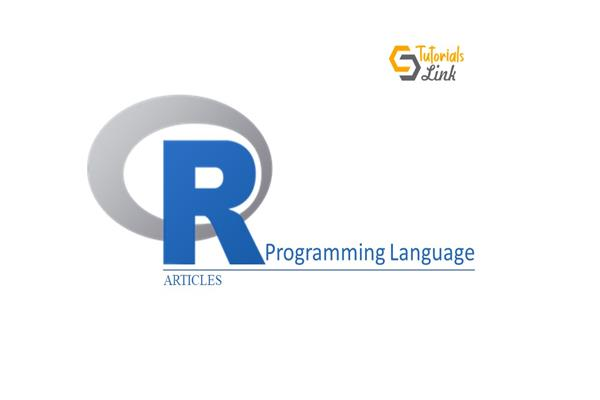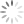Loading, please wait...What are the Operators in R programming Language?

Nov 23, 2021 #RLanguage #Programming, 171 Views

What are the Operators in the R programming language?

OPERATORS:

An operator is used to perform mathematical and logical operations on operands. R has all types of in-built operators that are available in other languages.

The different types of Operators in R Language:

• Assignment Operator
• Logical Operator
• Relational Operator
• Arithmetic Operator
• Miscellaneous Operator

Assignment Operator

Two operators fall under assignment operator:

1) Called Left Assignment (< -, =, << -)

Example:

vector1 <- c(8,4,TRUE,5+3i)

vector2 <<- c(8,4,TRUE,5+3i)

vector3 = c(8,4,TRUE,5+3i)

print(vector1)

print(vector2)

print(vector3)

OUTPUT

 8+0i 4+0i 1+0i 5+3i

 8+0i 4+0i 1+0i 5+3i

 8+0i 4+0i 1+0i 5+3i

2) Called Right Assignment(- >, - >>)

Example:

c(8,4,TRUE,5+3i) -> vector1

c(8,4,TRUE,5+3i) ->> vector2

print(vector1)

print(vector2)

OUTPUT

 8+0i 4+0i 1+0i 5+3i

 8+0i 4+0i 1+0i 5+3i

Logical Operator

The elements of the first vector are compared with the values of the second vector.

1) Element-wise logical AND Operator (&)

It compares the first element of the first vector with the values of the second vector. If both are true, it will return true otherwise false.

Example:

vector1 <- c(3,1,TRUE,2+3i)

vector2 <- c(4,1,FALSE,2+3i)

print(vector1&vector2)

OUTPUT

 TRUE TRUE FALSE TRUE

2) Element-wise logical OR Operator (|)

The comparison occurs between the values of the vectors. If any of the element value is true, it will return true otherwise false.

Example:

vector1 <- c(3,0,TRUE,2+2i)

vector2 <- c(4,0,FALSE,2+3i)

print(vector1|vector2)

OUTPUT

 TRUE FALSE TRUE TRUE

3) Logical NOT Operator (!)

It takes the individual elements of the vector and gives the opposite result.

Example

vector1 <- c(3,0,TRUE,2+2i)
print(!vector1)

OUTPUT

 FALSE TRUE FALSE FALSE

Relational Operator

The values of the first vector compare with the values of the second vector. It returns the output in the boolean expression.

1) Greater operator (>)

It checks whether the element of the first vector is greater than the second vector. If the value is greater, it will return true otherwise false.

Example

vector1 <- c(2,5.5,6,9)

vector2 <- c(8,2.5,14,9)

print(vector1>vector2)

OUTPUT

 FALSE TRUE FALSE FALSE

2) Lesser Operator(<)

It checks whether the element of the first vector is lesser than the second vector. If the value is lesser, it will return true otherwise false.

Example

vector1 <- c(2,5.5,6,9)

vector2 <- c(8,2.5,14,9)

print(vector1 < vector2)

OUTPUT

 TRUE FALSE TRUE FALSE

3) Equality Operator (==)

It checks the value of both the vectors is equal or not. If the values are equal, it will return true otherwise false.

Example

vector1 <- c(2,5.5,7,9)

vector2 <- c(3,2.5,7,9)

print(vector1 == vector2)

OUTPUT

 FALSE FALSE TRUE TRUE

4) Less than equal to Operator (<=)

It compares the element values of both the vector, whether they are lesser or equal. Returns true if the condition is true, otherwise false.

Example

vector1 <- c(2,5.5,6,9)

vector2 <- c(8,2.5,14,9)

print(vector1<=vector2)

OUTPUT

 TRUE FALSE TRUE TRUE

5) Greater than equal to Operator (>=)

It checks for the greater than or equal to condition. Return true if the condition is true, otherwise false.

Example

vector1 <- c(2,5.5,6,9)

vector2 <- c(8,2.5,14,9)

print(vector1>=vector2)

OUTPUT

 FALSE TRUE FALSE TRUE

Arithmetic Operator

An arithmetic operator performs operations such as addition, subtraction, multiplication, and more.

Example

v <- c( 2,5.5,6)

t <- c(8, 3, 4)

print(v+t)

OUTPUT

 10.0 8.5 10.0

2) Subtraction Operator

It subtracts two vectors.

Example

v <- c( 2,5.5,6)

t <- c(8, 3, 4)

print(v-t)

OUTPUT

 -6.0 2.5 2.0

Similarly, you can perform other arithmetic operations.

Miscellaneous Operator

These kinds of operators are used for a specific purpose.

1) Colon Operator (:)

It displays the sequence of the numbers.

Example

vector1 <- 2:8

print(vector1)

OUTPUT

 2 3 4 5 6 7 8

2) %in%

It is used to identify the existence of vectors in another vectors.

Example

vector1 <- 8

vector2 <- 12

vector3 <- 1:10

print(vector1 %in% vector3)

print(vector2 %in% vector3)

OUTPUT

 TRUE

 FALSE